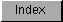```
After you have selected the desired interval between data samples, you can
calculate the value to place in the period register by using the period
formula:

desired interval     clock constant
Period value = ---------------- = ------------------
clock interval    samples per second

As an example, say you wanted to produce a 1 KHz sine wave, using a table
of eight data samples (four data words) (see Figure 5-3).

Figure 5-3: Example Sine Wave

Sampled Values:     0
90
127
90
0
-90
-127
-90

To output the series of eight samples at 1 KHz (1,000 cycles per second),
each full cycle is output in 1/1000th of a second. Therefore, each
individual value must be retrieved in 1/8th of that time. This translates
to 1,000 microseconds per waveform or 125 microseconds per sample. To
correctly produce this waveform, the period value should be:

125 microseconds/sample
Period value = ------------------------------ = 447 timing
0.279365 microseconds/interval   intervals/sample

To set the period register, you must write the period value into the
register AUDxPER, where "x" is the number of the channel you are using.
For example, the following instruction shows how to write a period value
of 447 into the period register for channel 0.

SETAUD0PERIOD:
LEA     CUSTOM,a0
MOVE.W  #447,AUD0PER(a0)

To produce high-quality sound, avoiding  aliasing distortion , you
should observe the limitations on period values that are discussed in
the section called "Producing Quality Sound."

For the relationship between period and musical pitch, see the section
at the end of the chapter, which contains a listing of the
equal-tempered musical scale .

```